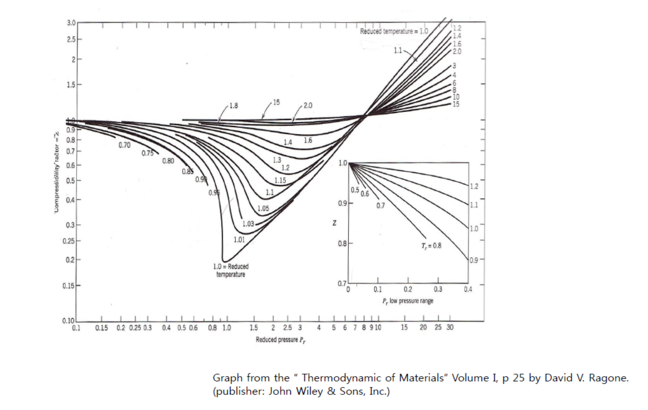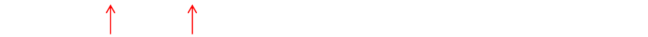2.12

# Equation of state for non-ideal gases

• The P-V-T relationship for real gas needs modification of ideal gas laws.
1) Compressibility factor
The compressibility factor Z is a correction term to the ideal gas laws.
PV=ZRT, z : compressibility factor
- Z can be estimated using the concept of “corresponding states”.
It states that gases behave similarly with respect to non-ideality;
that is, they have the same Z value when they are in corresponding
states relative to their respective critical pressure and critical
temperature.

- Thus, Z can be determined by knowing reduced pressure P$r$, and
reduced temperature T$r$.

- The Z values are tabulated (graphed) as a function of P$r$, T$r$, thus Z
depends on P, T.

$Pr=\frac{P}{Pc}, Tr=\frac{T}{Tc}$

where P, and T are the actual pressure and temperature and Pc
and Tc are the critical values.2) Van der Waals (vdw) equation of state
From ideal gas law, corrections in
① Volume
Real gas has finite volume (ideal gas = volume-less)
→ subtract the gas volume from the total volume.

② Another consideration
Particles are interacting each other. This correction should be     considered in the pressure term.

$(P$+$\frac {a}{V^2})$   $(V$$b)$=$RT$ $per$ $mole$